Surface Area and Volume : Exercise - 13.1 (Mathematics NCERT Class 10th)

Unless stated otherwise, take $\pi = {{22} \over 7}$

Q.1      2 cubes each of volume $64\,c{m^3}$ are joined end to end. Find the surface area of the resulting cuboid.
Sol.

Let  the  length  of each  edge of  the cube  be a cm.
Then, Volume  $= 64\,c{m^3}$
$\Rightarrow$  ${a^3} = 64$
$\Rightarrow$   a = 4

When two cubes of equal volumes (i.e., equal edges are joined end to end, we get a cuboid such that its.
l = Length = 4cm + 4cm = 8 cm
b = Breadth = 4 cm
and     h = Height = 4 cm
Therefore  Surface area of the cuboid
= 2(lb + bh + hl)
= 2(8 × 4 + 4 × 4 + 4 × 8) $c{m^2}$
= 2(32 + 16 + 32) $c{m^2}$
= (2 × 80) $c{m^2}$ = 160 $c{m^2}$

Q.2  A vessel is in the form of a hollow  hemisphere mounted by a hollow cylinder. The diameter of the  hemisphere is 14 cm and the total height of the vessel is 13 cm. Find the inner surface area of the vessel.
Sol.

Here r, the  radius of hemisphere  = 7 cm ,
h , the height  of cylinder = (13 – 7) cm = 6 cm
Clearly, radius of the base of cylindrical part is also r cm .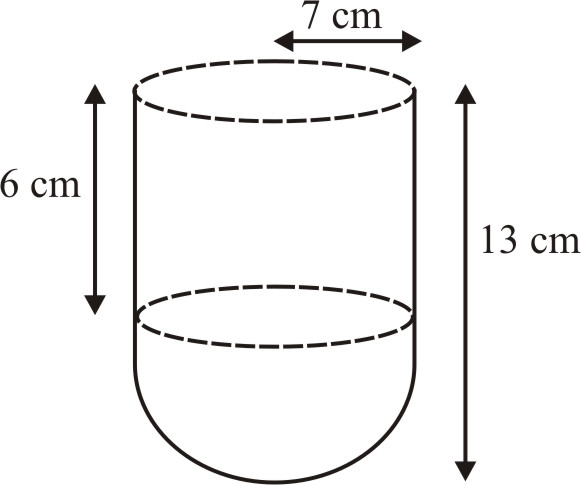Surface area of the vessel = Curved surface area of the cylindrical part + Curved surface area of hemispherical part
$= \left( {2\pi rh + 2\pi {r^2}} \right)c{m^2} = 2\pi r\left( {h + r} \right)c{m^2}$
$= 2 \times {{22} \over 7} \times 7 \times 13\,\,c{m^2} = 572\,c{m^2}$

Q.3  A toy is in the  form of a cone of radius 3.5 cm mounted on a hemisphere of same radius. The total  height of the toy is 15.5 cm. Find  the total surface area of the toy.
Sol.

We have VO = 15.5 cm,
OA = OO' = 3.5 cm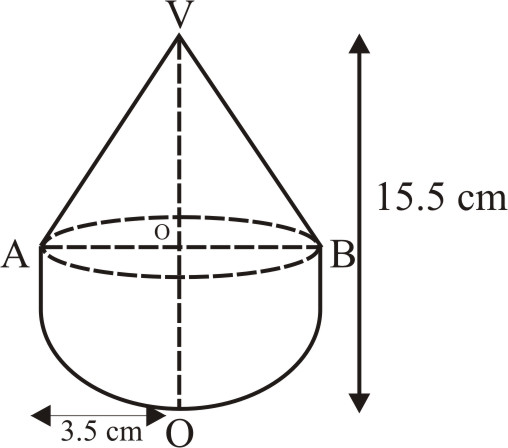Let r be the  radius of the base of cone and h be the height of conical part of the by.
Then r = OA = 3.5 cm
h = VO = VO' – OO = (15.5 – 3.5) cm = 12 cm
Also  radius  of the hemisphere = OA = r = 3.5 cm
Total surface area of the toy
= Curved surface area of cone + Curved surface area of hemisphere
$= \pi r\ell + 2\pi {r^2}$
where l = Slant height $= \sqrt {O{A^2} + O{V^2}} = \sqrt {{{\left( {3.5} \right)}^2} + {{12}^2}}$
$= \sqrt {12.25 + 144} = \sqrt {156.25} = 12.5\,cm$
$= \pi r\left( {\ell + 2r} \right)$
$= {{22} \over 7} \times 3.5 \times \left( {12.5 + 2 \times 3.5} \right)c{m^2}$
$= {{22} \over 7} \times 3.5 \times 19.5\,cm = 214.5\,\,c{m^2}$

Q.4  A cubical block  of side 7 cm is surmounted by a hemisphere. What is the greatest diameter of the  hemisphere can have? Find the surface area of the  solid?
Sol.

The  greatest diameter that a hemisphere  can have = 7 cm .
Surface area of the solid  after surmounted hemisphere
$= 6{\ell ^2} - \pi {R^2} + 2\pi {R^2}$
$= 6{\ell ^2} + \pi {R^2}$
$= 6{\left( 7 \right)^2} + {{22} \over 7} \times {\left( {{7 \over 2}} \right)^2}$
$= 6 \times 49 + 11 \times {7 \over 2}$
= 294 + 38.5  = 332.5 $c{m^2}$

Q.5  A hemispherical depression is cut  of  one face of a cubical wooden block  such the  diameter l of the  hemisphere is equal to the edge of the cube. Determine th surface area of the  remaining solid.
Sol.

Edge of the cube  = $\ell$
Diameter of the hemisphere  = $\ell$
Therefore  Radius  of the hemisphere = ${\ell \over 2}$
Therefore  Area  of the remaining  solid  after cutting out the  hemispherical  depression.
$= 6{\ell ^2} - \pi {\left( {{\ell \over 2}} \right)^2} + 2\pi {\left( {{\ell� \over 2}} \right)^2}$
$= 6{\ell ^2} + \pi {\left( {{\ell \over 2}} \right)^2}$
$= 6{\ell ^2} + \pi \times {{{\ell ^2}} \over 4} = {{{\ell ^2}} \over 4}\left( {24 + \pi } \right)$

Q.6  A medicine capsule is in the  shape of a cylinder with two hemispheres stuck to each of its ends (see figure). The length of the entire capsule is 14 mm and the  diameter of the  capsule is 5 mm. Find  its surface area.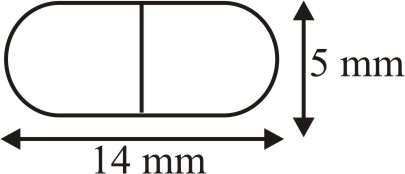Sol.

Let r cm  be the  radius and h cm  be the height  of the  cylinder. Then.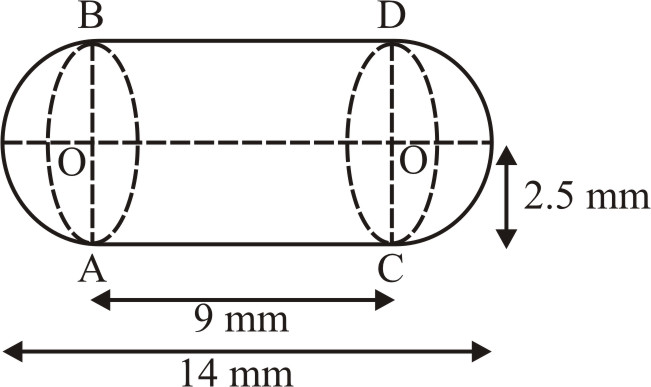$r = {5 \over 2}mm = 2.5\,mm$
and     $h = \left( {14 - 2 \times {5 \over 2}} \right)mm$
= (14 – 5)mm = 9mm
Also the  radius of hemisphere = ${5 \over 2}cm = r\,cm$
Now, surface area of the capsule = Curved surface of cylinder + Surface area of two hemispheres
$= \left( {2\pi rh + 2 \times 2\pi {r^2}} \right)m{m^2}$
$= 2\pi r\left( {h + 2r} \right)m{m^2}$
$= 2 \times {{22} \over 7} \times {5 \over 2} \times \left( {9 + 2 \times {5 \over 2}} \right)m{m^2}$
$= {{22} \over 7} \times 5 \times 14\,m{m^2} = 220\,m{m^2}$

Q.7 A tent is in the shape of a cylinder surmounted by a conical top. If the height and diameter of the  cylindrical part are 2.1 m and 4 m respectively, and the  slant height  of the top is 2.8 m, find  the area of the canvas used for making the tent. Also, find  the cost of the canvas of the tent at the rate of Rs 500 per ${m^2}$. (Note that the base of the tent will not covered with  canvas).
Sol.

We have, Total  canvas used  =  Curved surface area of cylinder + Curved surface area  of cone
$= \left( {2\pi rh + \pi r\ell } \right){m^2}$
$= \pi r\left( {2h + \ell } \right){m^2}$
$= {{22} \over 7} \times 2 \times \left( {2 \times 2.1 + 2.8} \right){m^2}$
$= {{22} \over 7} \times 2 \times 7\,{m^2}$
$= 44\,\,{m^2}$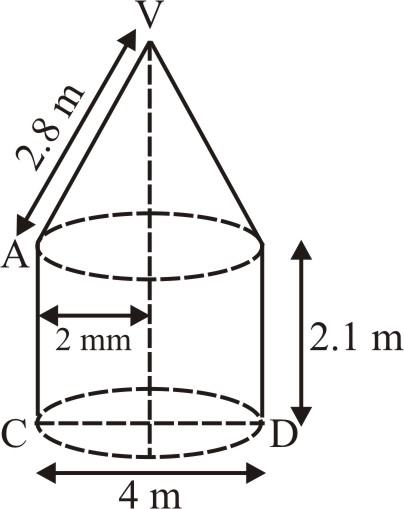Now,  cost of $1\,{m^2}$ the canvas for the  tent = Rs 500
So,  cost of $44\,{m^2}$ the  canvas for the  tent = Rs 44 × 500 = Rs 22000

Q.8 From  a solid  cylinder whose height  is 2.4 cm and diameter 1.4 cm, a conical  cavity of the same height  and same diameter is  hollowed out. Find the  total surface area of the remaining  solid to the  nearest $c{m^2}$.
Sol.

Radius  of the cylinder $= {{1.4} \over 2}cm = 7\,cm$
Height of the cylinder = 2.4 cm
Radius of the cone = .7 cm
Height  of the cone = 2.4 cm
Slant  height  of the cone
$= \sqrt {{{\left( {.7} \right)}^2} + {{\left( {2.4} \right)}^2}} \,cm$
$= \sqrt {0.49 + 5.76} \,cm$
$= \sqrt {6.25} \,cm = 2.5\,cm$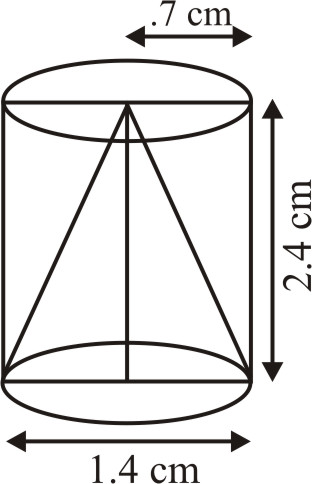Surface area of the remaining  solid = Curved surface area of cylinder + Curved surface of the  cone + Area of upper circular base of cylinder
$= 2\pi rh + \pi r\ell + \pi {r^2} = \pi r\left( {2h + \ell + r} \right)$
$= {{22} \over 7} \times .7 \times \left( {2 \times 2.4 + 2.5 + .7} \right)c{m^2}$
$= 2 \times .1 \times \left( {4.8 + 2.5 + .7} \right)c{m^2}$
$2.2 \times 8.0\,c{m^2} = 17.6\,c{m^2} = 18\,c{m^2}$

Q.9  A wooden article  was made by scooping out  a hemisphere  from each  end of a solid cylinder, as shown in figure. If the height  of the cylinder is 10 cm,and its base is of radius  3.5 m, find the  total  surface area of the article.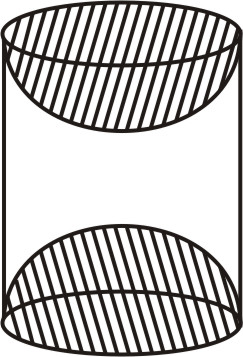Sol.

Surface area of the article when it is ready = Curved surface area of cylinder + 2 × Curved surface area of hemisphere.
$= 2\pi rh + 2 \times 2\pi {r^2}$
$= 2\pi r\left( {h + 2r} \right)$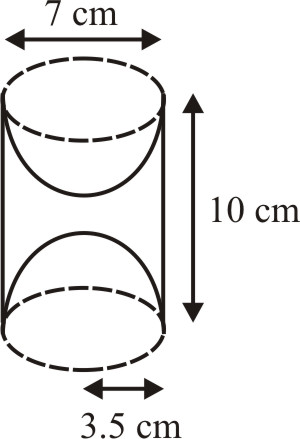where  r = 3.5 m and h = 10 cm
$= 2 \times {{22} \over 7} \times 3.5 \times \left( {10 + 2 \times 3.5} \right)c{m^2}$
$= 2 \times {{22} \over 7} \times 3.5 \times 17\,c{m^2} = 374c{m^2}$